# HSPT Math : How to do distance problems

## Example Questions

← Previous 1 3

### Example Question #1 : How To Do Distance Problems

Kate and Bella were both travelling at the same speed. Kate went 300 miles in 5 hours. Bella travelled 450 miles. How many hours did it take for Bella to reach her destination?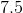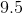Explanation:

The distance formula is essential in this problem.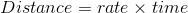First, use Kate's info to figure out the rate for both girls since they're travelling at the same speed, which is. Then, plug in that rate to the formula with Bella's information, which gives her a time ofhours.

### Example Question #11 : How To Solve Arithmetic Word Problems

Joe drove an average of 45 miles per hour along a 60-mile stretch of highway, then an average of 60 miles per hour along a 30-mile stretch of highway. What was his average speed, to the nearest mile per hour?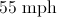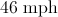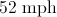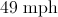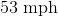Explanation:

At 45 mph, Joe drove 60 miles in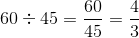hours.

At 60 mph, he drove 30 miles in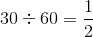hours.

He made the 90-mile trip in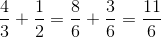hours, so divide 90 by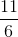to get the average speed in mph: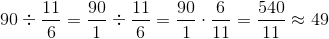### Example Question #1 : How To Do Distance Problems

1 mile = 5280 feet

If Greg's house is 5.3 miles away, how far is it in feet?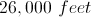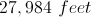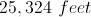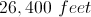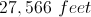Explanation:

Using the conversion formula, you would multiply 5.3 miles by 5280 feet and you will get 27,984 feet.

### Example Question #8 : Simplifying Expressions

Sophie travels f miles in g hours.  She must drive another 30 miles at the same rate.  Find the total number of hours, in terms of f and g, that the trip will take.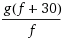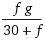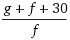g + f

g + f + 30Explanation:

Using d = rt, we know that first part of the trip can be represented by f = rg.  The second part of the trip can be represented by 30 = rx, where x is some unknown number of hours.  Note that the rate r is in both equations because Sophie is traveling at the same rate as mentioned in the problem.

Solve each equation for the time (g in equation 1, x in equation 2).

g = f/r

x = 30/r

The total time is the sum of these two times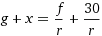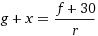Note that, from equation 1, r = f/g, so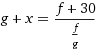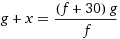=### Example Question #3 : How To Do Distance Problems

Gary is the getaway driver in a bank robbery. When Gary leaves the bank at 3 PM, he is going 60 mph, but the police officers are 10 miles behind traveling at 80 mph. When will the officers catch up to Gary?

3:10 PM

4:45 PM

5:00 PM

3:30 PM

4:00 PM

3:30 PM

Explanation:

Traveling 20 mph faster than Gary, it will take the officers 30 minutes to catch up to Gary. The answer is 3:30 PM.

### Example Question #1 : How To Do Distance Problems

Trevor took a road trip in his new VW Beetle. His car averages 32 miles per gallon. Gas costs \$4.19 per gallon on average for the whole trip. How much would it coust to drive 3,152 miles?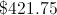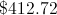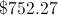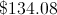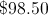Explanation:

To find this answer just do total miles divided by miles per gallon in order to find how many gallons of gas it will take to get from point A to Point B. Then multiply that answer by the cost of gasoline per gallon to find total amount spent on gasoline.

### Example Question #2 : Rate Problems

Jason is driving across the country. For the first 3 hours, he travels 60 mph. For the next 2 hours he travels 72 mph. Assuming that he has not stopped, what is his average traveling speed in miles per hour?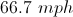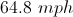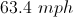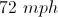Explanation:

In the first three hours, he travels 180 miles.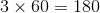In the next two hours, he travels 144 miles.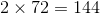for a total of 324 miles.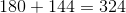Divide by the total number of hours to obtain the average traveling speed.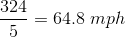### Example Question #221 : Problem Solving Questions

Tom runs a 100m race in a certain amount of time.  If John runs the same race, he takes 2 seconds longer.  If John ran at 8m/s, approximately how fast did Tom run?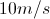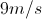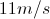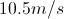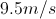Explanation:

Tom runs a 100m race in a certain amount of time.  If John runs the same race, he takes 2 seconds longer.  If John ran at 8m/s, how fast did Tom run?

Letdenote the amount of time that it took Tom to run the race.  Then it took John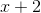seconds to run the same race going 8m/s.  At 8m/s, it takes 12.5 seconds to finish a 100m race.  This means it took Tom 10.5 seconds to finish.  Running 100m in 10.5 seconds is the same as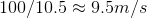### Example Question #5 : How To Do Distance Problems

Find the distance from point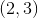to point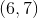.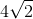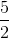Explanation:

Write the distance formula.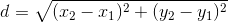Substitute the values of the points into the formula.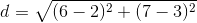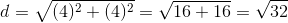The square root ofcan be reduced because, a factor of, is a perfect square.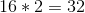Now we have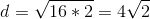### Example Question #1 : How To Do Distance Problems

Find the distance between the points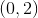and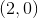.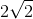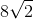Explanation:

Write the distance formula.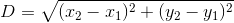Plug in the points.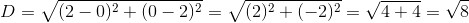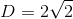The distance is:← Previous 1 3